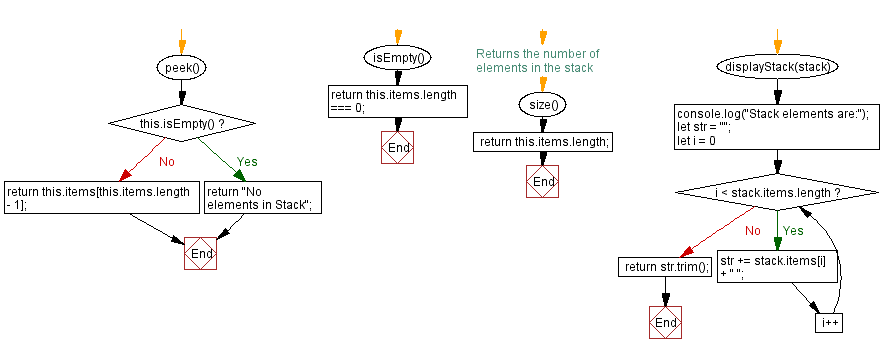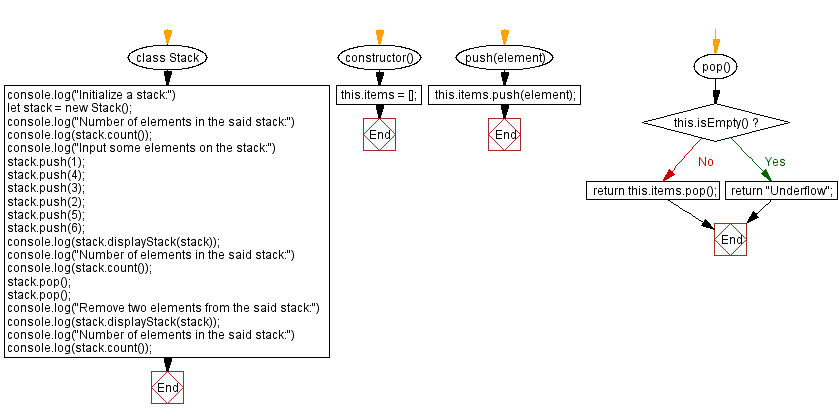# JavaScript Exercises: Count all the elements in a given stack

## JavaScript Stack: Exercise-7 with Solution

Write a JavaScript program to count all the elements in a given stack.

Sample Solution:

JavaScript Code:

``````class Stack {
constructor() {
this.items = [];
}
push(element) {
this.items.push(element);
}
pop() {
if (this.isEmpty())
return "Underflow";
return this.items.pop();
}

peek() {
if (this.isEmpty())
return "No elements in Stack";
return this.items[this.items.length - 1];
}
isEmpty() {
return this.items.length === 0;
}
// Returns the number of elements in the stack
size() {
return this.items.length;
}
displayStack(stack) {
console.log("Stack elements are:");
let str = "";
for (let i = 0; i < stack.items.length; i++)
str += stack.items[i] + " ";
return str.trim();
}
}
console.log("Initialize a stack:")
let stack = new Stack();
console.log("Number of elements in the said stack:")
console.log(stack.count());
console.log("Input some elements on the stack:")
stack.push(1);
stack.push(4);
stack.push(3);
stack.push(2);
stack.push(5);
stack.push(6);
console.log(stack.displayStack(stack));
console.log("Number of elements in the said stack:")
console.log(stack.count());
stack.pop();
stack.pop();
console.log("Remove two elements from the said stack:")
console.log(stack.displayStack(stack));
console.log("Number of elements in the said stack:")
console.log(stack.count());
```
```

Sample Output:

```"Initialize a stack:"
"Number of elements in the said stack:"
0
"Input some elements on the stack:"
"Stack elements are:"
"1 4 3 2 5 6"
"Number of elements in the said stack:"
6
"Remove two elements from the said stack:"
"Stack elements are:"
"1 4 3 2"
"Number of elements in the said stack:"
4
```

Flowchart:Live Demo:

* To run the code mouse over on Result panel and click on 'RERUN' button.*

Live Demo:

See the Pen javascript-common-editor by w3resource (@w3resource) on CodePen.

Improve this sample solution and post your code through Disqus

Stack Previous: Remove all the elements from a given stack.
Stack Exercises Next: Check if an element is present or not in a stack.

What is the difficulty level of this exercise?

Test your Programming skills with w3resource's quiz.

﻿